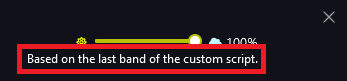# EO Browser Layer NDVI data is different from those obtained by the FIS query

I see the statistic of a polygon in EO Browser, but I can’t get the same answer via FIS Request. The results and dates do not match.
What Layer is used in the EO Browser for NDVI (custom scripts)?

Dear Iglw4,

is there any specific reason you are using a custom script and not the default visualization layer for the NDVI? If you use that layer, the correct statistics for the NDVI will be returned. I recommend you to use the prepared NDVI layer and check if the statistic for that is what you expect.

If you want to use a custom script to calculate the FIS (within EO Browser), you will see in the result that it says “Based on the last band of the custom script”.I assume that’s the difference you are experiencing is coming from that fact. If you want to get the correct statistics for the NDVI using a custom script, you can use the one below. It returns only 1 band (that holds the NDVI) and thus gives the correct statistic (note that here you don’t get the median/P10/P90 which is included in the default option).

``````function index(x, y) {
return (x - y) / (x + y);
}

function isCloud (scl) {
if (scl == 3) { // SC_CLOUD_SHADOW
return false;
} else if (scl == 9) { // SC_CLOUD_HIGH_PROBA
return true;
} else if (scl == 8) { // SC_CLOUD_MEDIUM_PROBA
return true;
} else if (scl == 7) { // SC_CLOUD_LOW_PROBA
return false;
} else if (scl == 10) { // SC_THIN_CIRRUS
return true;
} else if (scl == 11) { // SC_SNOW_ICE
return false;
} else if (scl == 1) { // SC_SATURATED_DEFECTIVE
return false;
} else if (scl == 2) { // SC_DARK_FEATURE_SHADOW
return false;
}
return false;
}

const cloud = isCloud (SCL)
const ndvi = index(B08, B04);
//return cloud ? [Number.NaN, 1] : [ndvi, 0];
return cloud ? [ndvi, 1] : [ndvi, 0];
``````

Best,
Daniel

Thank you so much Daniel…
Regards